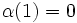# Coherent set of characters

Let$N$ be a normal subgroup of a finite group$G$. Let$S$ be a set of characters of$N$ (each character being a positive linear combination of irreducible characters). Let$\Z_0(S)$ denote the set of those integral linear combinations$\alpha$ of elements of$S$ such that$\alpha(1) = 0$.
We call$S$ a coherent set of characters if there exists a linear isometry$\tau$ from$\Z_0(S)$ to the character ring of$G$. Also$(S,\tau)$ is termed a coherent pair.